# PSEB 3rd Class Maths Solutions Chapter 8 Measurement

Punjab State Board PSEB 3rd Class Maths Book Solutions Chapter 8 Measurement Textbook Exercise Questions and Answers.

## PSEB Solutions for Class 3 Maths Chapter 8 Measurement

PAGE 162:
Let’s do:

Question 1.
Measure the length: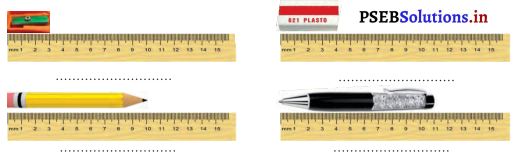Solution.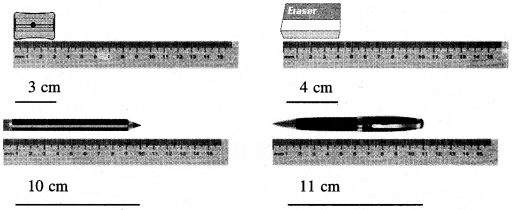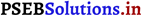PAGE 163:

Question 2.
Complete the following table by choosing some articles (things):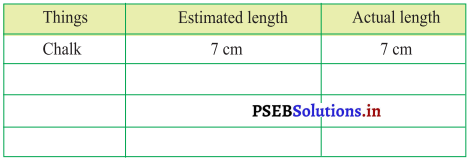Solution.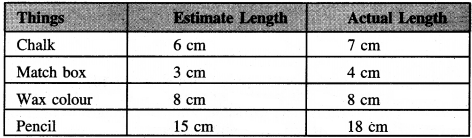Question 3.
Measure and write the length of the line segment in centimetres: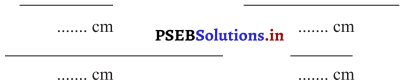Solution.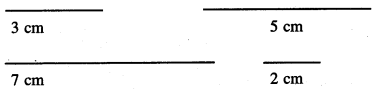PAGE 164:
Let’s do:

Draw the line segment of given length:
(a) 5 cm
(b) 8 cm
(c) 6 cm
(d) 10 cm
(e) 2 cm
(f) 7 cm
(g) 9 cm
(h) 12 cm
Sol.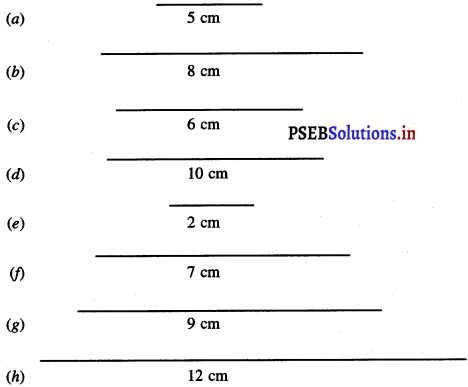Question 4.
Measure and write the length and breadth of real ₹ 100 currency note:
Length of the real ₹ 100 currency note _____ cm
Breadth of the real ₹ 100 currency note _____ cm
Solution.
Do it youself.

PAGE 165:
Let’s do:

Question 1.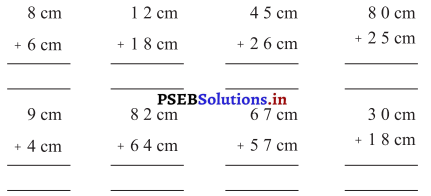Solution.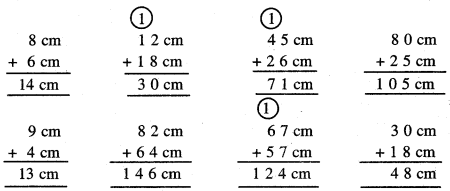Question 2.
Subtract: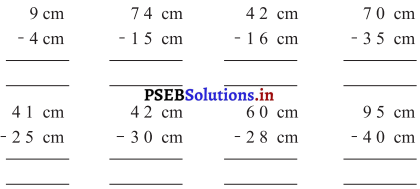Solution.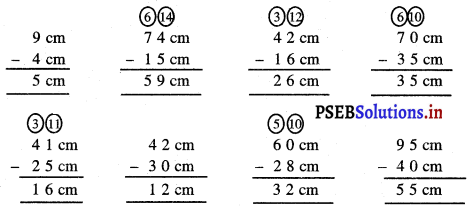Question 3.
In a school cloth will be given to girl students. 2 metre cloth will be given to Simran for shirt and 3 metre will be given for salwar. Find how much cloth in total Simran requires for uniform.
Solution.
Cloth required for shirt of Simran = 2 m
Cloth required for salwar of Simran = 3 m
Total cloth required for her uniform = 5 m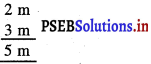Question 4.
Satveer’s school is 175 m away from her house. Find the distance which she covers from her house to her school and from her school to her house ?
Solution.
Distance of school from Satveer’s house = 175 m
Distance of Satveer’s house from school = 175 m
Distance covered by her in one day from house to school and from school to house = 350m.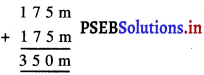Question 5.
Saroj used 60 cm long red ribbon and 75 cm long green ribbon to make a flower. What is the total length of ribbon used by Saroj?
Solution.
Length of red ribbon used = 75 cm
Length of green ribbon used = 60 cm
Total length of ribbon used = 135 cm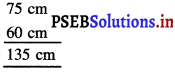So, 135 cm ribbon is used to make flower.PAGE 166:

Weight:

Do you remember?

Question 1.
There is a geometry box in your one hand while a pencil in your other hand, which thing is heavier?
Solution.
Geometry box

Question 2.
There is a pappaya in your one hand while there is an apple in the other, which of these is heavier?
Solution.
Papaya

Question 3.
There are 20 mangoes in one basket while another basket has 20 berries. Which basket is heavier?
Solution.

PAGE 168:
Let’s do:

Question 1.
Show the position of the pointer according to the weight of the objects: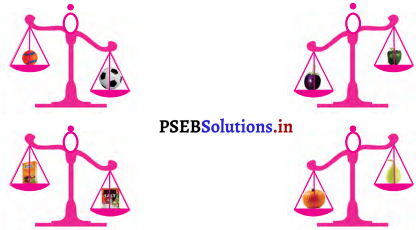Solution.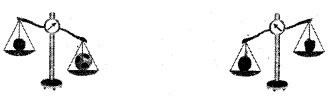Question 2.
Find heavier, lighter or equal.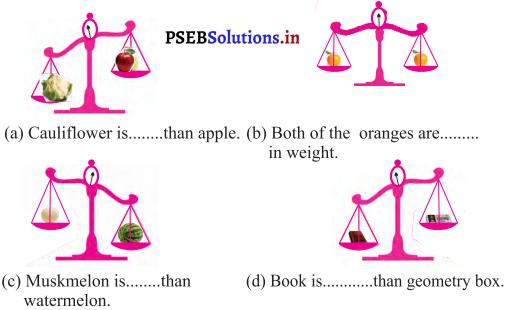Solution.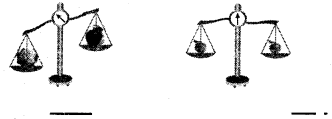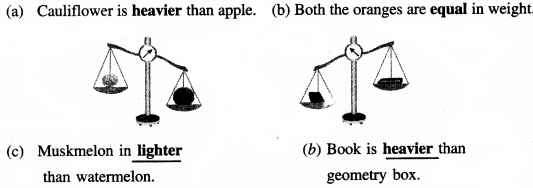Question 3.
Write the following things in light weight to heavy weight order (1 to 5) based on their weight: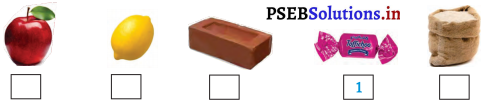Solution.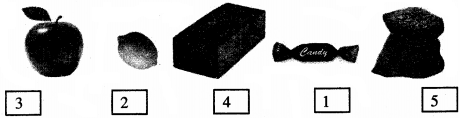Question 4.
One brick weighs 3 kg. Write the things in following table those arc heavier or lighter than the brick.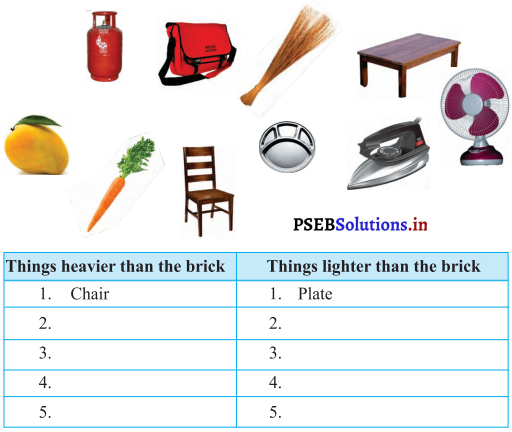Solution.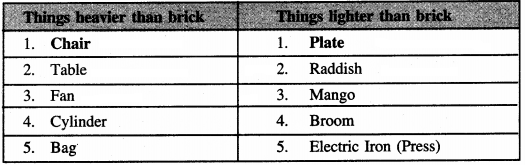PAGE 171:
Let’s do:

Question 1.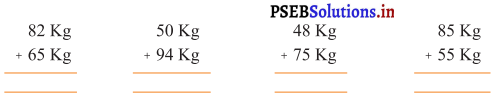Solution.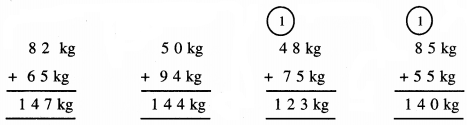Subtract: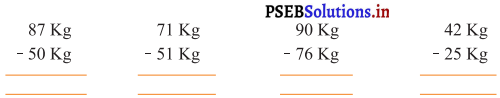Solution.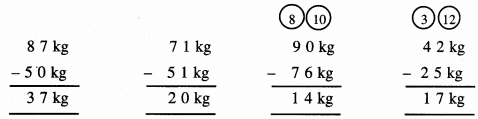Question 3.
Amrik Singh bought 25 kg flour and 8 kg sugar. He bought how many kg of grocery ?
Solution.
Amrik bought flour = 25 kg
Amrik bought sugar = 8 kg
Total ration = 33 kg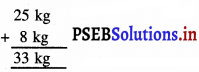So, Amrik bought total ration of 33 kg.

Question 4.
Vegetable seller Shanker bought 90 kg potatoes from vegetable market. He sold 42 kg potatoes. How much potatoes are left with him ?
Solution.
Quantity of Potatoes bought = 90 kg
Quantity of Potatoes sold = 42 kg
Remaining Potatoes left with him = 48 kg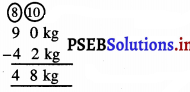So, 48 kg potatoes left with him.Question 5.
In a Govt, school 103 kg wheat and 98 kg rice are used in mid-day meal during one month. How much grains is used in school during one month ?
Solution.
Wheat used in mid-day meal in one month = 103 kg
Rice used in mid day meal in one month = 98 kg
Total grains used in mid day meal during one month = 201 kg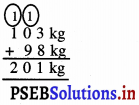So, 201 kg of grains is used during one month.

PAGE 172:
Do you remember?

Capacity:

The capacity of a bottle is equal to the 3 glasses of water then :
(a) Capacity of 2 bottles will be equal to the _____ glasses of water.
Solution.
6

(b) Capacity of 3 bottles will be equal to the _____ glasses of water.
9

(c) Capacity of 4 bottles will be equal to the _____ glasses of water.
Solution.
12

(d) Capacity of 5 bottles will be equal to the _____ glasses of water.
Solution.
15

(e) Capacity of 6 bottles will be equal to the _____ glasses of water.
Solution.
18PAGE 174:
Let’s do:

Write the following objects as 1 to 5 as per their capacity: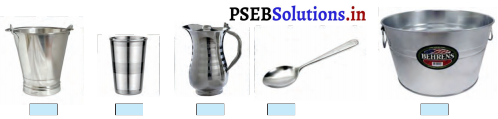Solution.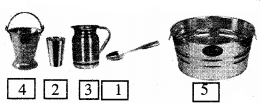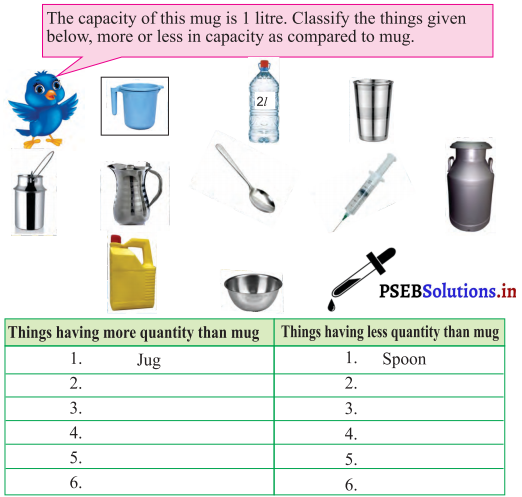Solution.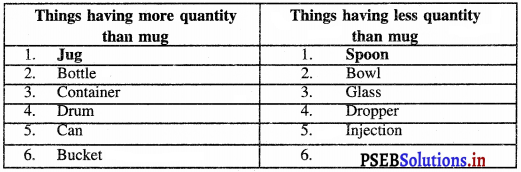PAGE 175:

Find less, more or equal: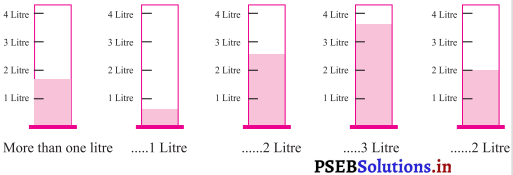Solution.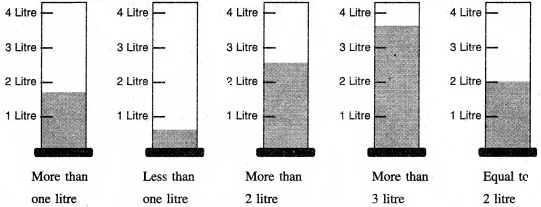Colour the vessels according to given capacity: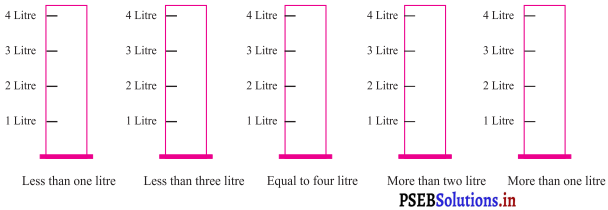Solution.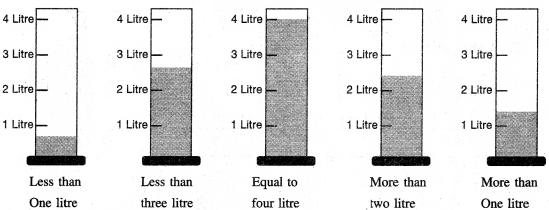Question 5.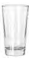Fill some vessels with the help of a glass glasses are needed to fill each of them: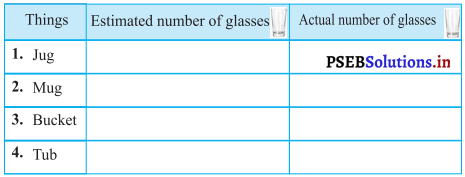Solution.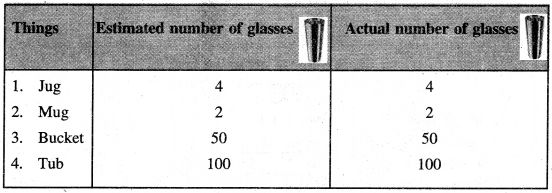Question 6.
Find the capacity of above vessels with the help of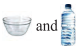.
Solution.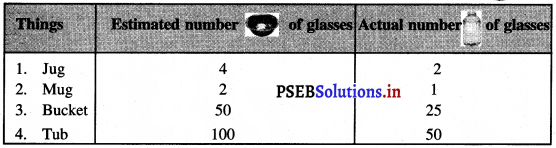PAGE 176:
Lets do:

Question 1.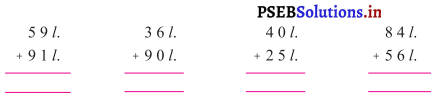Solution.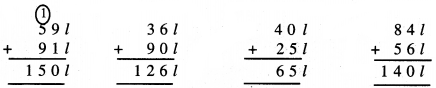Question 2.
Subtract: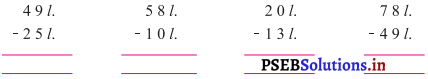Solution.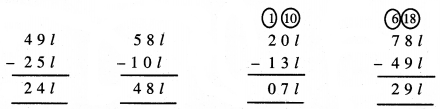PAGE 177:

Question 3.
Smt. Devki Rani bought 5 litre of refined oil and 2 litre of mustard oil for her house. How much oil did she buy?
Solution.
Refined oil bought by Devki Rani = 5 l
Mustard oil bought by Devki Rani = 2 l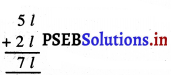Devki Rani bought 7 litre of oil = 7 l

Question 4.
One family consumes 3751 of water in a day. If the capacity of water tank is 500 l then how much water is left in the tank?
Solution.
Water in tank = 500 l
Water used = 375 l
Water left in tank = 125 l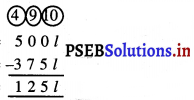125 litres of water is left in water tank.Worksheet:

Question 1.
How many centimetres are there in one metre ?
(a) 10 cm
(b) 100 cm
(c) 1000 cm
(d) 10,000 cm
Solution.
(b) 100 cm

Question 2.
How many centimetres are there in 4 metres ?
(a) 300 cm
(b) 4000 cm
(c) 400 cm m
(d) 40 cm
Solution.

Question 3.
The length of pencil is 18 cm and a length of piece of chalk is 6 cm. How much is the pencil longer than the piece of a chalk ?
(a) 18 cm
(b) 16 cm
(c) 12 cm
(d) 14 cm
Solution.
(c) 12 cmQuestion 4.
The length of pen is 12 cm and sharpner’s length is 2 cm. How much is the pen longer than the sharpner ?
(a) 8 cm
(b) 6 cm
(c) 9 cm
(d) 10 cm
Solution.
(d) 10 cm

Question 5.
Raman’s mother bought 5 kg potatoes and 4 kg onions from a vegetable shop. What is total quantity she bought ?
(a) 7 kg
(b) 9 kg
(c) 12 kg
(d) 8 kg
Solution.
(b) 9 kg

Question 6.
A sweet seller made 20 kg laddoo’s in one day and sold 10 kg of laddoos out of it. How much quantity of laddoos was left with him?
(a) 7 kg
(b) 10 kg
(c) 20 kg
(d) 25 kg
Solution.
(b) 10 kgQuestion 7.
A vessel contains 10 litres of milk and another vessel contains 15 litres of milk. What is total quality of milk in both the vessels?
(a) 25 l
(b) 15 l
(c) 10 l
(d) 35 l
Solution.
(a) 25 l

Question 8.
Which of the following is measured in litres?
(a) Wood
(b) petrol
(c) Cloth
(d) Book
Solution.
(b) petrolQuestion 9.
Who use the balance?
(a) Teacher
(b) Vegetable seller
(e) Hair Dresser
(d) Washer man.
Solution.
(b) Vegetable seller